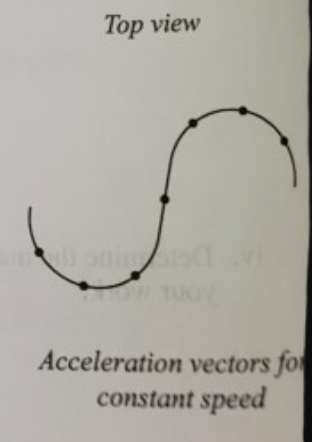# Problem: Suppose that an object were to move at constant speed around an S-shaped curve as shown at right. The S-shaped curve is made of two half circles and a straight segment.At each of the indicated points on the diagram, draw a vector to represent the acceleration of the object. If the magnitude of the acceleration is zero at any of these points, indicate so explicitly.

###### FREE Expert Solution

Acceleration is directed towards the center of the circular path.

90% (373 ratings)###### Problem Details

Suppose that an object were to move at constant speed around an S-shaped curve as shown at right. The S-shaped curve is made of two half circles and a straight segment.

At each of the indicated points on the diagram, draw a vector to represent the acceleration of the object. If the magnitude of the acceleration is zero at any of these points, indicate so explicitly.